Try NerdPal! Our new app on iOS and Android

# Solve the differential equation $dx+e^{3x}dy=0$

## Related Videos

Go!
Go!
1
2
3
4
5
6
7
8
9
0
a
b
c
d
f
g
m
n
u
v
w
x
y
z
.
(◻)
+
-
×
◻/◻
/
÷
2

e
π
ln
log
log
lim
d/dx
Dx
|◻|
θ
=
>
<
>=
<=
sin
cos
tan
cot
sec
csc

asin
acos
atan
acot
asec
acsc

sinh
cosh
tanh
coth
sech
csch

asinh
acosh
atanh
acoth
asech
acsch

### Videos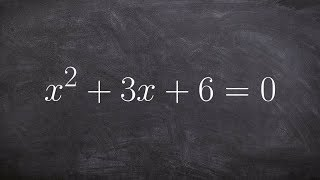### Algebra 2 - Learn how solve a quadratic by completing the square with fractions, x^2 + 3x + 6 = 0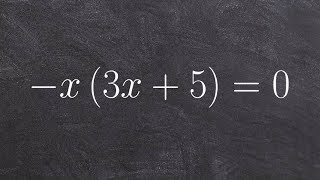### Algebra 2 - Solving a factored quadratic equation using the zero product property, -x(3x + 5) = 0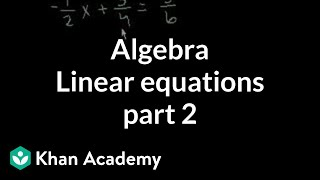### Algebra: Linear equations 2 | Linear equations | Algebra I | Khan Academy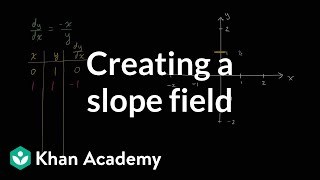### Creating a slope field | First order differential equations | Khan Academy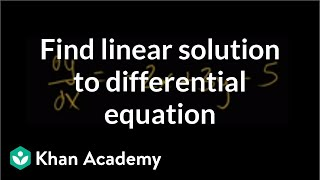### Finding particular linear solution to differential equation | Khan Academy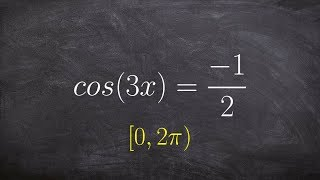SnapXam A2

### beta Got another answer? Verify it!

Go!
1
2
3
4
5
6
7
8
9
0
a
b
c
d
f
g
m
n
u
v
w
x
y
z
.
(◻)
+
-
×
◻/◻
/
÷
2

e
π
ln
log
log
lim
d/dx
Dx
|◻|
θ
=
>
<
>=
<=
sin
cos
tan
cot
sec
csc

asin
acos
atan
acot
asec
acsc

sinh
cosh
tanh
coth
sech
csch

asinh
acosh
atanh
acoth
asech
acsch

$dx+e^{3x}dy=0$